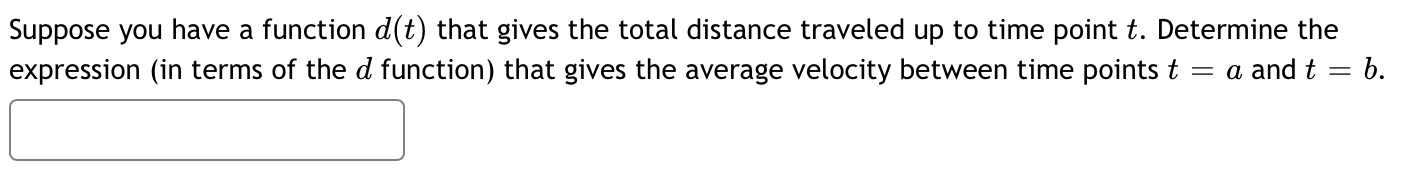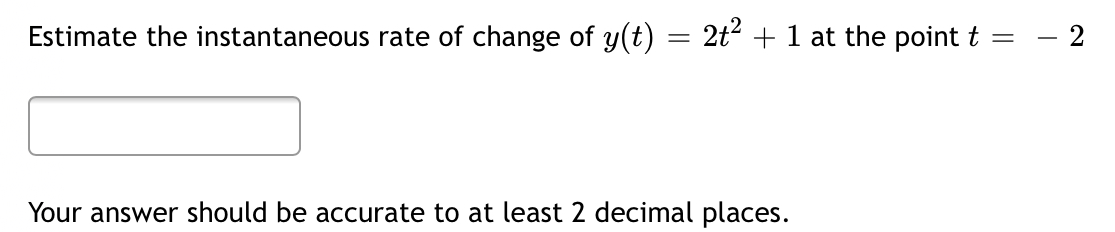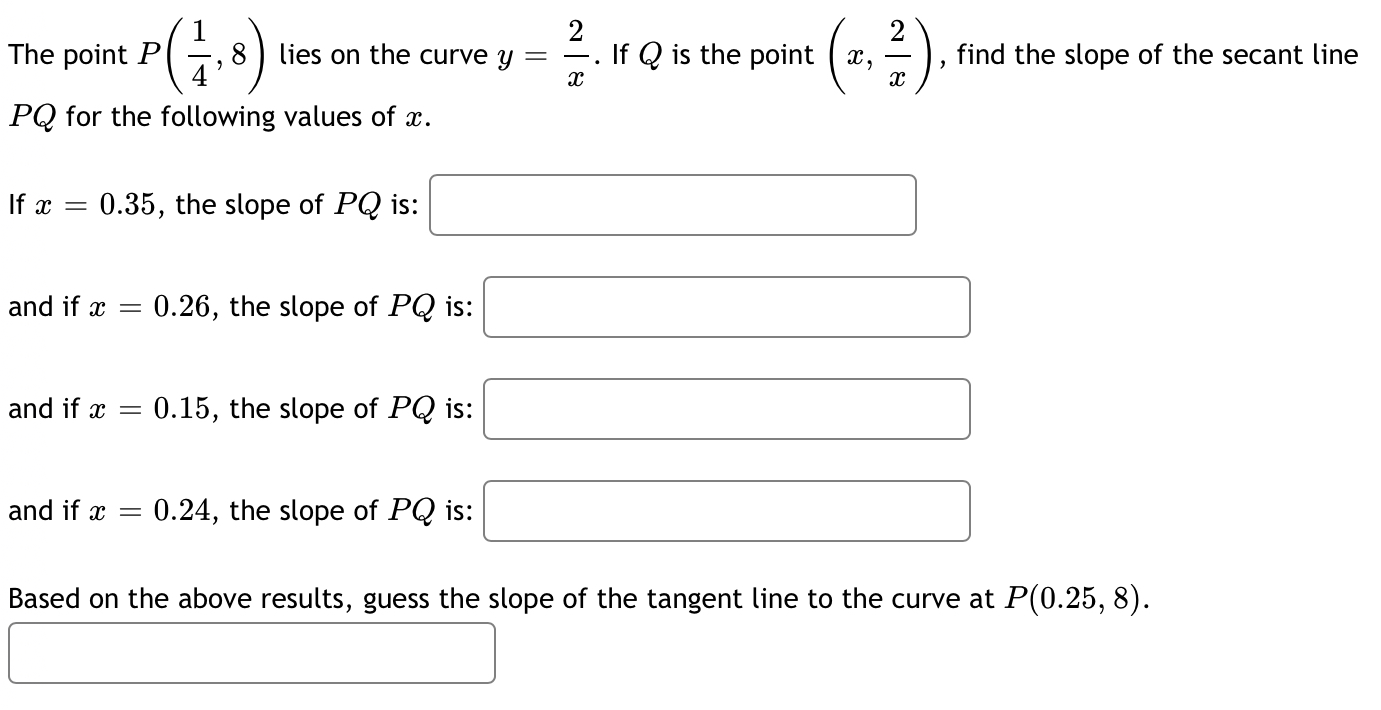# Question Solved1 Answer1. 2. 3. Suppose you have a function d(t) that gives the total distance traveled up to time point t. Determine the expression (in terms of the d function) that gives the average velocity between time points t = a and t b. Estimate the instantaneous rate of change of y(t) 2t2 + 1 at the point t = 2 Your answer should be accurate to at least 2 decimal places. 2. The point P (18) lies on the curve y = If Q is the point ( x, (2, 4). find the slope of the secant line C PQ for the following values of x. If x = 0.35, the slope of PQ is: and if x = 0.26, the slope of PQ is: and if x = 0.15, the slope of PQ is: and if x = 0.24, the slope of PQ is: Based on the above results, guess the slope of the tangent line to the curve at P(0.25, 8).1.2.3.Transcribed Image Text: Suppose you have a function d(t) that gives the total distance traveled up to time point t. Determine the expression (in terms of the d function) that gives the average velocity between time points t = a and t b. Estimate the instantaneous rate of change of y(t) 2t2 + 1 at the point t = 2 Your answer should be accurate to at least 2 decimal places. 2. The point P (18) lies on the curve y = If Q is the point ( x, (2, 4). find the slope of the secant line C PQ for the following values of x. If x = 0.35, the slope of PQ is: and if x = 0.26, the slope of PQ is: and if x = 0.15, the slope of PQ is: and if x = 0.24, the slope of PQ is: Based on the above results, guess the slope of the tangent line to the curve at P(0.25, 8).
More
Transcribed Image Text: Suppose you have a function d(t) that gives the total distance traveled up to time point t. Determine the expression (in terms of the d function) that gives the average velocity between time points t = a and t b. Estimate the instantaneous rate of change of y(t) 2t2 + 1 at the point t = 2 Your answer should be accurate to at least 2 decimal places. 2. The point P (18) lies on the curve y = If Q is the point ( x, (2, 4). find the slope of the secant line C PQ for the following values of x. If x = 0.35, the slope of PQ is: and if x = 0.26, the slope of PQ is: and if x = 0.15, the slope of PQ is: and if x = 0.24, the slope of PQ is: Based on the above results, guess the slope of the tangent line to the curve at P(0.25, 8).